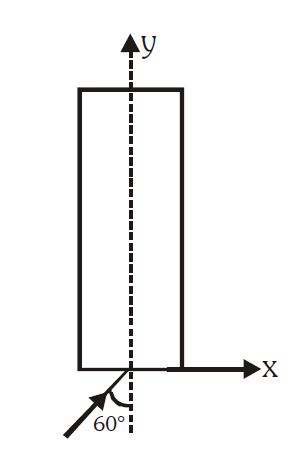Maximum value of Y coordinate!A ray of light enters into a thick glass slab from air as shown in figure. The refractive index varies as $\mu =(2\sqrt3 - y)$.

If width of slab is very large then maximum value of $y$-coordinate of the ray is?

×